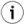### 18.5.6.Functions by Category

#### General Box Functions

The box, usually marked with the caddr_t data type is the basic representation of any SQL data in Virtuoso. All boxes have a run time data type, with a name beginning with `DV_` . All boxes have a 3 byte run time length which allows for up to 16 MB of contiguous array size in SQL data.

The further interpretation of the content of the box is determined by the type tag. The length is always an exact byte length, although the actual length is rounded up to the next suitably aligned value. The length and tag of a box must never be changed while the box is allocated but the content is freely writable. The tag and length reside immediately under the pointer of the box, so that a box, with the appropriate type cast will pass as a C array or string.

Numbers are generally represented as boxes. There is an exception for small integers, which are always distinguishable from pointers. Thus the range from -10000 to 10000 are not allocated as boxes holding the value but can be passed directly. This is hidden however and the programmer need not be concerned about this except sometimes when debugging.

The byte order in boxes depends on the platform.

The most important types are:

 DV_SHORT_STRING - The box contains a null terminated string of char. The box length is 1 + the count of characters, including the final 0 in the count. DV_LONG_STRING - same as DV_SHORT_STRING. DV_LONG_INT, TAG_BOX - The box is sizeof (long) long, with the long as element 0, in the appropriate byte order. DV_SINGLE_FLOAT - sizeof (float) bytes, containing the float. DV_DOUBLE_FLOAT - sizeof (double) bytes, contains the double. DV_NUMERIC - opaque, contains a decimal floating point. The `numeric_t` functions can be used, see appropriate documentation. DV_BIN - Binary string, no terminating 0 counted in the length. DV_DATETIME - datetime, opaque, dt_ functions can be used, see appropriate documentation. DV_BLOB_HANDLE - Blob handle, opaque but convertible to string if short enough. DV_ARRAY_OF_POINTER - Heterogeneous array, sizeof (caddr_t) * n_elements, first all elements are box pointers. The length is in bytes, so divide by sizeof (caddr_t). DV_DB_NULL - a box of 0 bytes (header only), represents the SQL NULL value.

#### Box Functions

 `box_t dk_alloc_box (` uint32 bytes , int tag `)`;

`dk_alloc_box()` allocates a box of the given size and type. The initial contents are undefined.

 `int dk_free_box (` box_t box `)`;

`dk_free_box()` frees a box allocated by `dk_alloc_box()` . The argument may not be any other pointer.

 `int dk_free_tree (` box_t box `)`;

`dk_free_tree()` is like `dk_free_box()` but will free recursively, following through DV_ARRAY_OF_POINTER boxes.

 `uint32 box_length (` box_t box2 `)`;
```#define box_tag(box) \
(*((dtp_t *) &(((unsigned char *)(box))[-1])))
```

These return the length and the tag of a box.

 `long unbox (` box_t n `)`;
 `box_t box_num (` long n `)`;
 `box_t box_dv_short_string (` char *string `)`;
 `box_t box_double (` double d `)`;
 `box_t box_float (` float f `)`;
```#define unbox_num(n) unbox(n)
#define unbox_float(f) (*((float *)f))
#define unbox_double(f) (*((double *)f))
#define unbox_string(s) ((char *)s)
```

The above functions and macros convert between C data types and boxes. `box_dv_short_string()` takes a `char *` to any null terminated string and allocates a string box of appropriate size. This itself looks like a null terminated string but has the box header with the run time length and type under the pointer.

 `box_t box_copy (` box_t box `)`;

`box_copy()` returns an identical size box with the same type and contents.

 `box_t box_copy_tree (` box_t box `)`;

`box_copy_tree()` performs a recursive copy, traversing `DV_ARRAY_OF_POINTER` references.

 `int box_equal (` box_t b1 , box_t b2 `)`;

Given two arbitrary boxes, returns true if they are recursively equal.

Example18.2.Box Examples

Below is the code for box_copy_tree:

```box_t
box_copy (box_t box)
{
dtp_t tag;
uint32 len;
box_t copy;

if (!IS_BOX_POINTER (box))
return box;

tag = box_tag (box);
if (box_copier[tag])
return (box_copier[tag] (box));
len = box_length (box);
copy = dk_alloc_box (len, tag);
memcpy (copy, box, (uint32) len);
return copy;
}
```
```box_t
box_copy_tree (box_t box)
{
box_t *copy;
dtp_t tag;

if (!IS_BOX_POINTER (box))
return box;

tag = box_tag (box);
copy = (box_t *) box_copy (box);
if (tag == DV_ARRAY_OF_POINTER || tag == DV_LIST_OF_POINTER)
{
uint32 inx, len = BOX_ELEMENTS (box);
for (inx = 0; inx < len; inx++)
copy[inx] = box_copy_tree (((box_t *) box)[inx]);
}

return (box_t) copy;
}
```

Note:The `IS_BOX_POINTER` check at the start will detect the unboxed, 'bare' small integers which are actually not allocated and can be returned by value. Only then can box_tag be used to find the type. The DV_TYPE_OF macro should be used instead of box_tag when the type is unknown to avoid de-referencing a small integer. Also note `BOX_ELEMENTS` , which is box_length () / sizeof (caddr_t). This is practical for iterating over arrays.
See AlsoThe VSEI Functions .